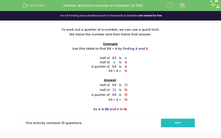# Divide by 4 Using Halving and Halving Again

In this worksheet, students will work out a quarter of a number by halving and halving again.Key stage:  KS 2

Curriculum topic:   Number: Multiplication and Division

Curriculum subtopic:   Use Multiplication/Division Facts (3, 4 and 8)

Difficulty level:#### Worksheet Overview

In this activity, we will be learning a method of dividing by 4 to find one quarter of a number.

One quarter means 1 out of 4 parts.

So to find one quarter of a number, we need to divide it into 4 equal parts, as shown in this bar model.To work out a quarter of a number quickly, we can use a mental method to divide by 4.

First, we halve the number.

This diagram shows how to use this method to find one quarter of 24.

Half of  24  is
 12
Half of
 12
is
 6
A quarter of  24  is
 6
24 ÷ 4 =
 6

Let's try an example question together.

Example

Use this table to find 64 ÷ 4 by finding A and B.

Half of  64  is
 A
Half of
 A
is
 B
A quarter of  64  is
 B
64 ÷ 4 =
 B

First, we find half of 64. This is A.

A is worth 32.

Next, we find half of A (32), which is 16.

Half of  64  is
 32
Half of
 32
is
 16
A quarter of  64  is
 16
64 ÷ 4 =
 16

So A is 32 and B is 16.

One quarter of 64 is 16.Now that you know this method, it's time to try dividing some numbers by 4.

Good luck!

### What is EdPlace?

We're your National Curriculum aligned online education content provider helping each child succeed in English, maths and science from year 1 to GCSE. With an EdPlace account you’ll be able to track and measure progress, helping each child achieve their best. We build confidence and attainment by personalising each child’s learning at a level that suits them.

Get started